# Montel theorem

Jump to: navigation, search

Montel's theorem on the approximation of analytic functions by polynomials: Ifis an open set in the complex-plane not containing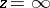andis a single-valued function, analytic at each point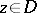, then there is a sequence of polynomials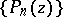converging toat each. This theorem is one of the basic results in the theory of approximation of functions of a complex variable; it was obtained by P. Montel .

Montel's theorem on compactness conditions for a family of holomorphic functions (principle of compactness, see ): Letbe an infinite family of holomorphic functions in a domainof the complex-plane, thenis pre-compact, that is, any subsequence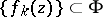has a subsequence converging uniformly on compact subsets of, ifis uniformly bounded in. This theorem can be generalized to a domainin,(see Compactness principle).

Montel's theorem on conditions for normality of a family of holomorphic functions (principle of normality, see ): Let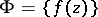be an infinite family of holomorphic functions in a domainof the complex-plane. If there are two distinct valuesandthat are not taken by any of the functions, thenis a normal family, that is, any sequencehas a sequence uniformly converging on compact subsets ofto a holomorphic function or to. The conditions of this theorem can be somewhat weakened: It suffices that all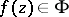do not take one of the values, say, and that the other valueis taken at mosttimes,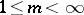. This theorem can be generalized to a domainin,.